# AP Calculus AB : Comparing relative magnitudes of functions and their rates of change

## Example Questions

← Previous 1 3 4 5 6 7 8 9 10 11

### Example Question #1 : Comparing Relative Magnitudes Of Functions And Their Rates Of Change

Evaluate the definite integral of the algebraic function.

integral (x+ √(x))dx from 0 to 1

11/12

0

5/12

1

10/12

11/12

Explanation:

Step 1: Rewrite the problem.

integral (x3+x1/2) dx from 0 to 1

Step 2: Integrate

x4/4 + 2x(2/3)/3 from 0 to 1

Step 3: Plug in bounds and solve.

[14/4 + 2(1)(2/3)/3] – [04/4 + 2(0)(2/3)/3] = (1/4) + (2/3) = (3/12) + (8/12) = 11/12

### Example Question #2 : Comparing Relative Magnitudes Of Functions And Their Rates Of Change

Evaluate the integral.

Integral from 1 to 2 of (1/x3) dx

1/2

–3/8

0

–5/8

3/8

3/8

Explanation:

Integral from 1 to 2 of (1/x3) dx

Integral from 1 to 2 of (x-3) dx

Integrate the integral.

from 1 to 2 of (x–2/-2)

(2–2/–2) – (1–2/–2) = (–1/8) – (–1/2)=(3/8)

### Example Question #3 : Comparing Relative Magnitudes Of Functions And Their Rates Of Change

Evaluate the following indefinite integral.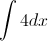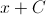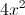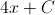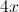Explanation:

In order to evaluate the indefinite integral, ask yourself, "what expression do I differentiate to get 4".  Next, use the power rule and increase the power ofby 1. To start, we have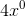, so in the answer we have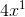.  Next add a constant that would be lost in the differentiation.  To check your work, differentiate your answer and see that it matches "4".

### Example Question #4 : Comparing Relative Magnitudes Of Functions And Their Rates Of Change

Evaluate the following indefinite integral.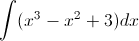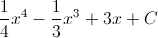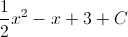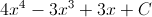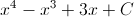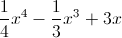Explanation:

Use the inverse Power Rule to evaluate the integral.  We know that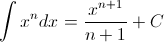for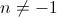.  We see that this rule tells us to increase the power ofby 1 and multiply by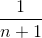.  Next always add your constant of integration that would be lost in the differentiation.  Take the derivative of your answer to check your work.

### Example Question #5 : Comparing Relative Magnitudes Of Functions And Their Rates Of Change

Evaluate the following indefinite integral.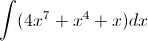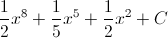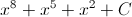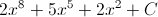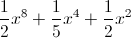Explanation:

Use the inverse Power Rule to evaluate the integral.  We know thatfor.  We see that this rule tells us to increase the power ofby 1 and multiply by.  Next always add your constant of integration that would be lost in the differentiation.  Take the derivative of your answer to check your work.

### Example Question #6 : Comparing Relative Magnitudes Of Functions And Their Rates Of Change

Evaluate the following indefinite integral.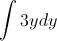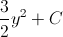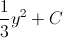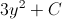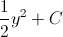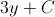Explanation:

Use the inverse Power Rule to evaluate the integral.  Firstly, constants can be taken out of integrals, so we pull the 3 out front.  Next, according to the inverse power rule, we know thatfor.  We see that this rule tells us to increase the power ofby 1 and multiply by. Next always add your constant of integration that would be lost in the differentiation.  Take the derivative of your answer to check your work.

### Example Question #7 : Comparing Relative Magnitudes Of Functions And Their Rates Of Change

Evaluate the following indefinite integral.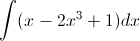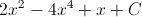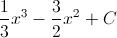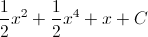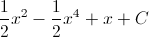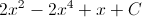Explanation:

Use the inverse Power Rule to evaluate the integral.  We know thatfor.  We see that this rule tells us to increase the power ofby 1 and multiply by.  Next always add your constant of integration that would be lost in the differentiation.  Take the derivative of your answer to check your work.

### Example Question #8 : Comparing Relative Magnitudes Of Functions And Their Rates Of Change

Evaluate the following indefinite integral.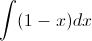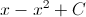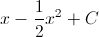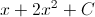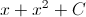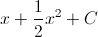Explanation:

Use the inverse Power Rule to evaluate the integral.  We know thatfor.  We see that this rule tells us to increase the power ofby 1 and multiply byNext always add your constant of integration that would be lost in the differentiation.  Take the derivative of your answer to check your work.

### Example Question #9 : Comparing Relative Magnitudes Of Functions And Their Rates Of Change

Evaluate the following indefinite integral.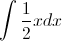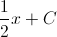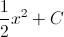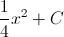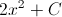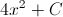Explanation:

Use the inverse Power Rule to evaluate the integral.  Firstly, constants can be taken out of the integral, so we pull the 1/2 out front and then complete the integration according to the rule. We know thatfor.  We see that this rule tells us to increase the power ofby 1 and multiply by.  Next always add your constant of integration that would be lost in the differentiation.  Take the derivative of your answer to check your work.

### Example Question #3 : Comparing Relative Magnitudes Of Functions And Their Rates Of Change

Evaluate the following indefinite integral.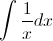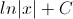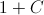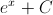Use the inverse Power Rule to evaluate the integral.  We know thatfor. But, in this case,IS equal toso a special condition of the rule applies.  We must instead use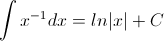.  Evaluate accordingly.  Next always add your constant of integration that would be lost in the differentiation.  Take the derivative of your answer to check your work.